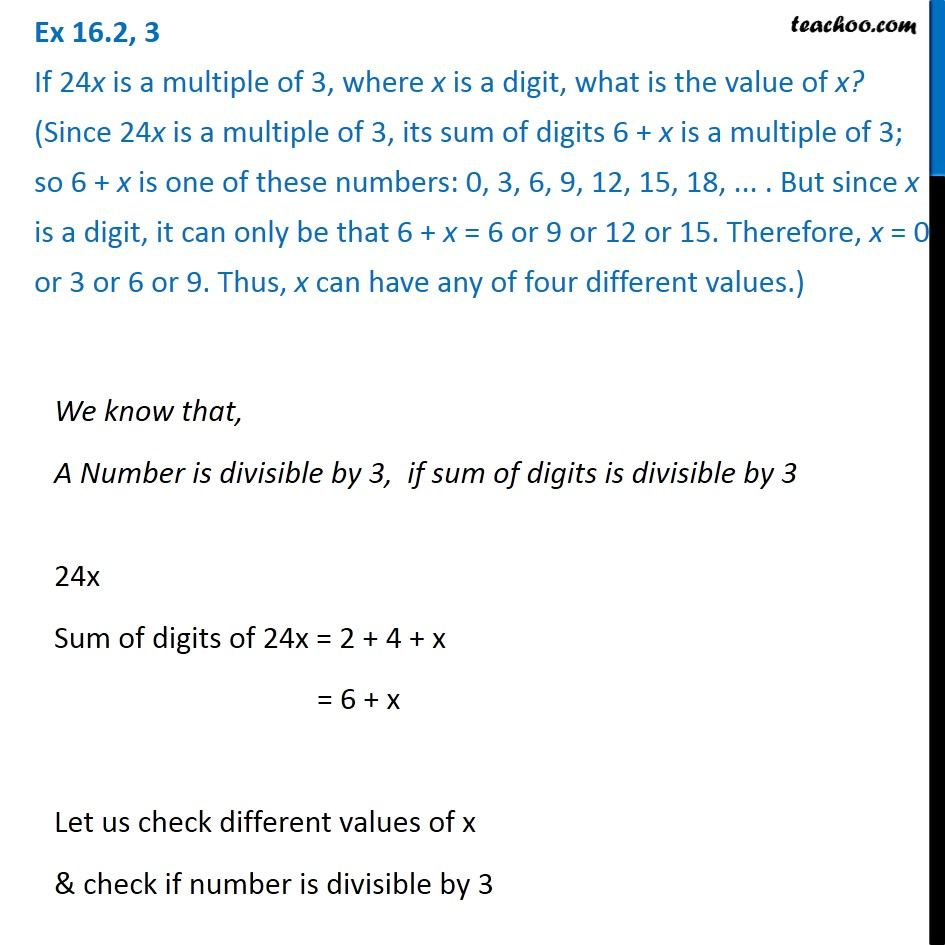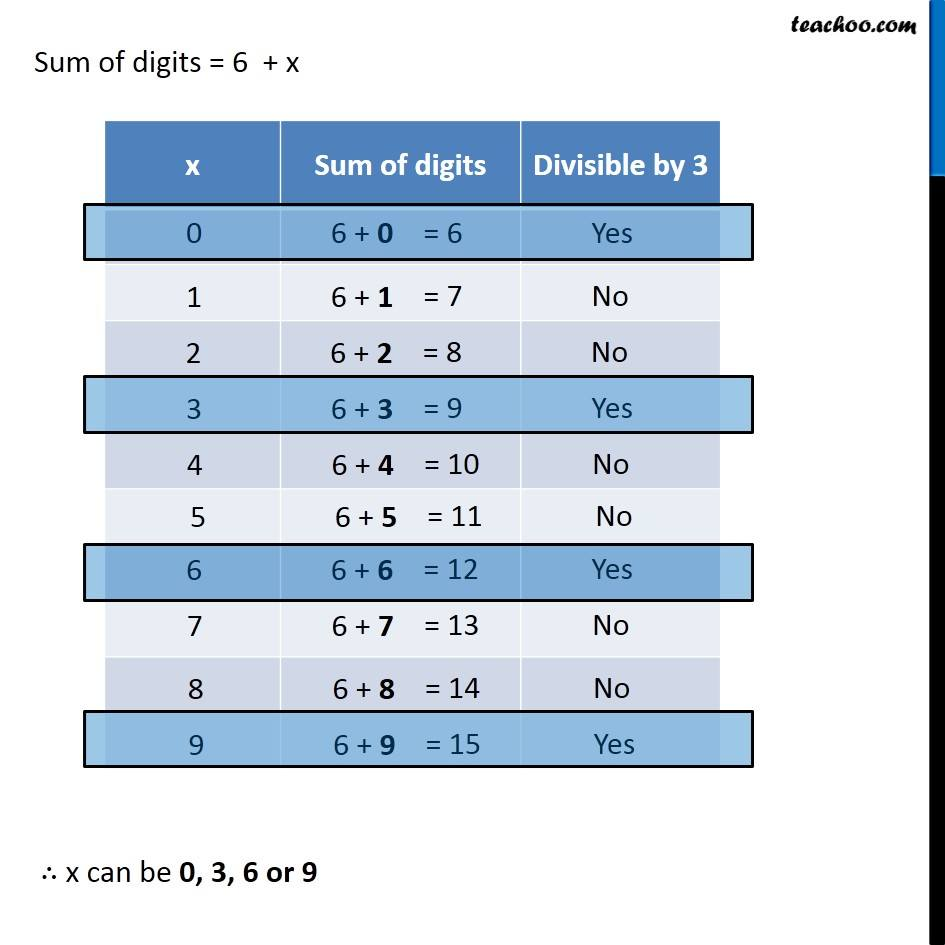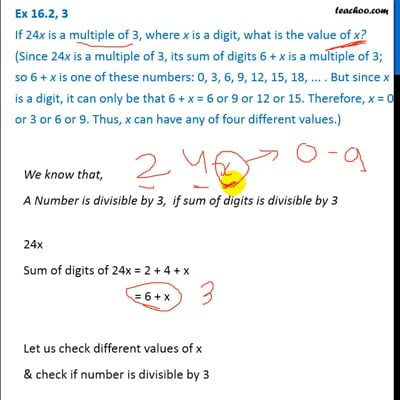Tests of Divisibility

Chapter 16 Class 8 Playing with Numbers
Concept wiseThis video is only available for Teachoo black users

Solve all your doubts with Teachoo Black (new monthly pack available now!)

### Transcript

Ex 16.2, 3 If 24x is a multiple of 3, where x is a digit, what is the value of x? (Since 24x is a multiple of 3, its sum of digits 6 + x is a multiple of 3; so 6 + x is one of these numbers: 0, 3, 6, 9, 12, 15, 18, ... . But since x is a digit, it can only be that 6 + x = 6 or 9 or 12 or 15. Therefore, x = 0 or 3 or 6 or 9. Thus, x can have any of four different values.)We know that, A Number is divisible by 3, if sum of digits is divisible by 3 24x Sum of digits of 24x = 2 + 4 + x = 6 + x Let us check different values of x & check if number is divisible by 3 Sum of digits = 6 + x ∴ x can be 0, 3, 6 or 9